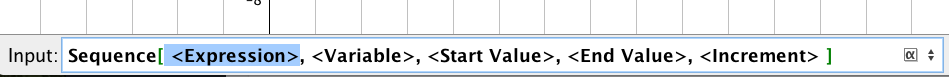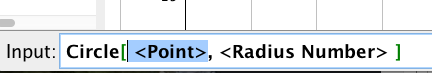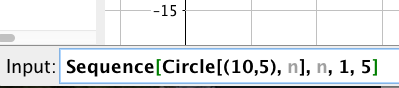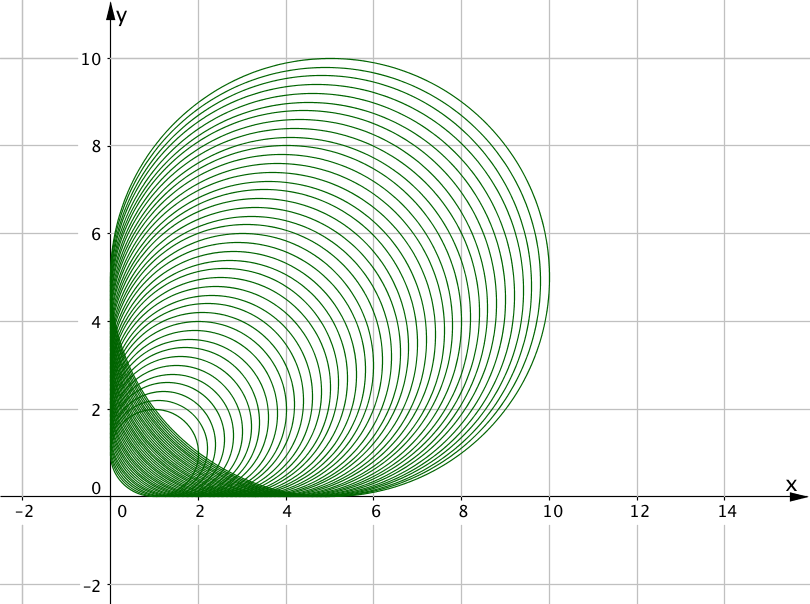# The Sequence Command

The sequence command of geogebra, combined with other commands, yields some fun diagrams!

The sequence command:Let’s make a sequence of points, with coordinates, for values ofbetweenand. That is,To generate this sequence of points, we type in:Which yields the graph:You can try that out on the geogebra applet below. You  many be able to copy, paste this command:

Sequence[(n,n^2),n,1,5,1]

# Sequences of Circles

Now suppose we want to draw a sequence of circles. The circle command requires a centre and a radius.Suppose we make a sequence of circles with centreand with radius.

Try out the following command in the geogebra applet below:To copy paste use: Sequence[Circle[(10,5),n],n,1,5]

# Geogebra applet:

If that worked for you, try out

Sequence[Circle[(10,5), n], n, 1, 5, 0.1]

The final parameter changes the increment offrom the default value 1 to 0.1. More steps between 1 and 5 – more circles.

# Make some of your own

Use the geogebra applet to generate your own sequence of circles.

1. Make a sequence where the centre of the circle is constant, but the radius changes.
2. Make a sequence where the centre of the circle changes, but the radius is constant.
3. Make a sequence where the centre and the radius both change.

# Figure out the sequence command

Now see if you can reproduce the following diagrams:

### Project 1### Project 2### ProJect 3### Solutions

1. Sequence[Circle[(n, n), n], n, 1, 5, 0.1]
2. Sequence[Circle[(n, 5), n], n, 1, 5, 0.05]
3. Sequence[Circle[(12 + n, 5), n], n, 1, 5, 0.05]

# Sequences of Line Segments

The following diagram uses the command Segment[<point>,<point>] to create a sequence of line segments. Observe the start/end point to a few of line segments to see the pattern of start and end points.